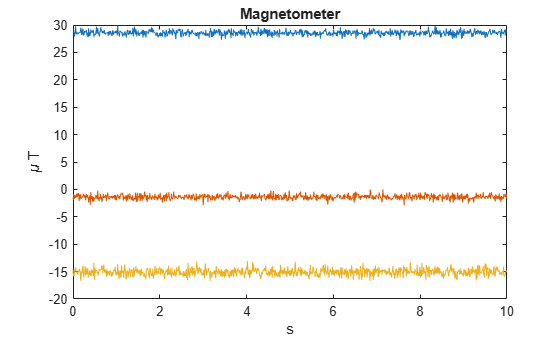# magparams

Magnetometer sensor parameters

## Description

The magparams class creates a magnetometer sensor parameters object. You can use this object to model a magnetometer when simulating an IMU with imuSensor. See the Algorithms section of imuSensor for details of magparams modeling.

## Creation

### Syntax

params = magarams
params = magparams(Name,Value)

### Description

params = magarams returns an ideal magnetometer sensor parameters object with default values.

params = magparams(Name,Value) configures magparams object properties using one or more Name,Value pair arguments. Name is a property name and Value is the corresponding value. Name must appear inside single quotes (''). You can specify several name-value pair arguments in any order as Name1,Value1,...,NameN,ValueN. Any unspecified properties take default values.

## Properties

expand all

Maximum sensor reading in μT, specified as a real positive scalar.

Data Types: single | double

Resolution of sensor measurements in μT/LSB, specified as a real nonnegative scalar. Here, LSB is the acronym for least significant bit.

Data Types: single | double

Constant sensor offset bias in μT, specified as a real scalar or 3-element row vector. Any scalar input is converted into a real 3-element row vector where each element has the input scalar value.

Data Types: single | double

Sensor axes skew in percentage, specified as a scalar, a 3-element row vector, or a 3-by-3 matrix with values ranging from 0 to 100. The diagonal elements of the matrix account for the misalignment effects for each axes. The off-diagonal elements account for the cross-axes misalignment effects. The measured state vmeasure is obtained from the true state vtrue via the misalignment matrix as:

${v}_{measure}=\frac{1}{100}M\text{ }{v}_{true}=\frac{1}{100}\left[\begin{array}{ccc}{m}_{11}& {m}_{12}& {m}_{13}\\ {m}_{21}& {m}_{22}& {m}_{23}\\ {m}_{31}& {m}_{32}& {m}_{33}\end{array}\right]{v}_{true}$

• If you specify the property as a scalar, then all the off-diagonal elements of the matrix take the value of the specified scalar and all the diagonal elements are 100.

• If you specify the property as a vector [a b c], then m21 = m31 = a, m12 = m32 = b, and m13 = m23 = c. All the diagonal elements are 100.

Data Types: single | double

Power spectral density of sensor noise in μT/√Hz, specified as a real scalar or 3-element row vector. Any scalar input is converted into a real 3-element row vector where each element has the input scalar value.

Data Types: single | double

Instability of the bias offset in μT, specified as a real scalar or 3-element row vector. Any scalar input is converted into a real 3-element row vector where each element has the input scalar value.

Data Types: single | double

Integrated white noise of sensor in (μT/√Hz), specified as a real scalar or 3-element row vector. Any scalar input is converted into a real 3-element row vector where each element has the input scalar value.

Data Types: single | double

Sensor bias from temperature in (μT/℃), specified as a real scalar or 3-element row vector. Any scalar input is converted into a real 3-element row vector where each element has the input scalar value.

Data Types: single | double

Scale factor error from temperature in (%/℃), specified as a real scalar or 3-element row vector with values ranging from 0 to 100. Any scalar input is converted into a real 3-element row vector where each element has the input scalar value.

Data Types: single | double

## Examples

collapse all

Generate magnetometer data for an imuSensor object from stationary inputs.

Generate a magnetometer parameter object with a maximum sensor reading of 1200 $\mu T$ and a resolution of 0.1 $\mu T/LSB$. The constant offset bias is 1 $\mu T$. The sensor has a power spectral density of$\text{\hspace{0.17em}}\left(\frac{\left[0.6\text{\hspace{0.17em}}\text{\hspace{0.17em}}0.6\text{\hspace{0.17em}}\text{\hspace{0.17em}}0.9\right]}{\sqrt{100}}\right)$ $\mu T/\sqrt{Hz}$. The bias from temperature is [0.8 0.8 2.4] $\mu T/{}^{0}C$. The scale factor error from temperature is 0.1 %$/{}^{0}C$.

params = magparams('MeasurementRange',1200,'Resolution',0.1,'ConstantBias',1,'NoiseDensity',[0.6 0.6 0.9]/sqrt(100),'TemperatureBias',[0.8 0.8 2.4],'TemperatureScaleFactor',0.1);

Use a sample rate of 100 Hz spaced out over 1000 samples. Create the imuSensor object using the magnetometer parameter object.

Fs = 100; numSamples = 1000; t = 0:1/Fs:(numSamples-1)/Fs; imu = imuSensor('accel-mag','SampleRate', Fs, 'Magnetometer', params);

Generate magnetometer data from the imuSensor object.

orient = quaternion.ones(numSamples, 1); acc = zeros(numSamples, 3); angvel = zeros(numSamples, 3); [~, magData] = imu(acc, angvel, orient);

Plot the resultant magnetometer data.

plot(t, magData) title('Magnetometer') xlabel('s') ylabel('\mu T')## Version History

Introduced in R2018b